Courses

# Design of Cyclones Computer Science Engineering (CSE) Notes | EduRev

## Computer Science Engineering (CSE) : Design of Cyclones Computer Science Engineering (CSE) Notes | EduRev

The document Design of Cyclones Computer Science Engineering (CSE) Notes | EduRev is a part of the Computer Science Engineering (CSE) Course Environmental Engineering.
All you need of Computer Science Engineering (CSE) at this link: Computer Science Engineering (CSE)

DESIGN OF CYCLONES

Cyclone separators utilizes a centrifugal forces generated by a spinning gas stream to separate the particulate matters from the carrier gas. The centrifugal force on particles in a spinning gas stream is much greater than gravity; therefore cyclones are effective in the removal of much smaller particles than gravitational settling chambers, and require much less space to handle the same gas volumes. In operation, the particle-laden gas upon entering the cyclone cylinder receives a rotating motion. The vortex so formed develops a centrifugal force, which acts to particle radially towards the wall. The gas spirals downward to the bottom of the cone, and at the bottom the gas flow reveres to form an inner vortex which leaves through the outlet pipe .

Theory

In a cyclone, the inertial separating force is the radial component of the simple centrifugal force and is a function of the tangential velocity. The centrifugal force can be expressed by Fc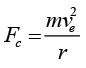( 2.3.1)
Where, m=mass of the particle, ve=tangential velocity of the particle at radius r, and r=radius of rotation. The separation factor S is given by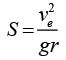(2.3.2)
The separation factor varies from 5 in large, low velocity units to 2500 in small, high pressure units. Higher the separation factor better is the performance of the cyclone. In the cyclone, the gas, in addition to moving in a circular path, also moves radially inwards between the inlet on the periphery and the exit on the axis. Since the tangential velocities of the particle and the gas are the same, the relative velocity between the gas and particle is simply equal to the radial velocity of the gas. This result in a drag force on the particle towards the centre, and the equilibrium radius of rotation of the particle can be obtained by balancing the radial drag force and the centrifugal force: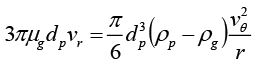(2.3.3)

Where, dp=particle diameter, and vr=radial velocity of the gas at radius r. Arranging the above equation, for vr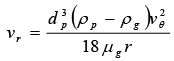2.3.4)
The tangential velocity of the particle in the vortex has been found experimentally to be inversely proportional to the radius of rotation according to equation,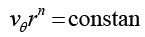(2.3.5)

Where, n is the exponent and dimensionless. For an ideal gas n=1. The real values observed are between 0.5 to 1, depending upon the radius of the cyclone body and gas temperature. Vθ can be related to the tangential velocity at the inlet to the cyclone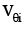as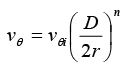(2.3.6)
Where, D=diameter of the cyclone.may be taken as the velocity of the gas through the inlet pipe, i.e.,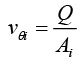(2.3.7)
Where, Q=gas volumetric flow rate and Ai=cross-sectional area of the inlet. Therefore,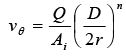(2.3.8)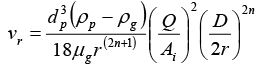(2.3.9)

The most satisfactory expression for cyclone performance is still the empirical one. Lapple correlated collection efficiency in terms of the cut size dpe which is the size of those particle that are collected with 50% efficiency. Particle larger than dpe will have collection efficiency greater than 50% while the smaller particle will be collected with lesser efficiency. The cut size is given by: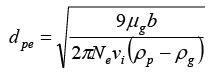(2.3.10)

Where, b=inlet width, vi=gas inlet velocity and Ne=effective number of turns a gas makes in traversing the cyclone (5 to 10 in most cases).

Pressure drop: The pressure drop may be estimated according to the following equation,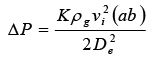(2.3.11 )
Where, K=a constant, which averages 13 and ranges from 7.5 to 18.4, ΔP=pressure drop, a, b and De=cyclone dimensions, vi=inlet gas velocity and ρg =gas density.

Problem 2.3.1: A conventional cyclone with diameter 0.5 m handles 4.0 m3/s of standard air (µg=1.81×10-5 kg/m-s and ρg being negligible w.r.t ρp) carrying particles with a density of 2500 kg/m3. For Ne=6, inlet width (b)=0.25 m, inlet height (a)=0.5 m, determine the cut size of particle diameter.

Solution: Given b=0.25,  D=0.25×0.5=0.1 a=0.5,   D=0.5×0.5=0.25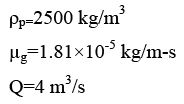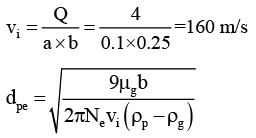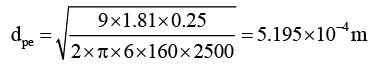Offer running on EduRev: Apply code STAYHOME200 to get INR 200 off on our premium plan EduRev Infinity!

## Environmental Engineering

14 videos|73 docs|70 tests

,

,

,

,

,

,

,

,

,

,

,

,

,

,

,

,

,

,

,

,

,

;